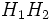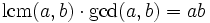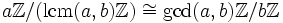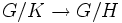# Understanding the isomorphism theorems

Jump to: navigation, search
YOU MAY ALSO BE INTERESTED IN: Understanding the definition of a homomorphism, Understanding the quotient map, Understanding the notions of order and index

The isomorphism theorems are basic results about the way homomorphisms and quotient maps behave for groups, and how they interact with intersections, products and other operations involving groups. This article looks at the isomorphism theorems from a number of perspectives, concentrating on some of the key aspects.

## The natural choice aspect

### Explicit isomorphism, rather than just an assertion of isomorphism

A naive interpretation of the isomorphism theorems is to think of them as simply saying that certain groups, constructed in certain ways, are isomorphic. However, this does not capture the power of the theorems. The theorems explicitly construct isomorphisms that are the natural choice for such an isomorphism; in all cases, the isomorphism constructed by the theorem is effectively the only possible thing to write down.

The map that describes the isomorphism is the real power of the result, rather than the fact that the groups happen to be isomorphic.

### The left and right analogues

All the isomorphism theorems are stated with respect to certain assumptions of normality, but they all have analogues, often slightly weaker formulations, when the subgroups involved are not normal. These analogues have both left and right versions -- by the natural equivalence of left and right in groups (via the inverse map) the left and right formulations are equivalent.

The formulations involving non-normal subgroups, however, do not talk about isomorphisms between groups -- they talk of natural bijections between sets. What the isomorphism theorems tell us is that these bijections between sets become isomorphisms between groups when the subgroups involved are normal.

Analogous to the normal subgroups case, the map that describes the bijection is the real power of the result, rather than the fact that the fact that the sets have equal cardinalities.

In this article we shall review each isomorphism theorem, its left analogue for non-normal subgroups, and its many implications. The article starts with the second isomorphism theorem; the first isomorphism theorem is covered in the survey article understanding the quotient map.

## The second isomorphism theorem

### The version for non-normal subgroups: the product formula

Further information: product formula

Suppose$H_1, H_2$ are subgroups of a group$G$. Let$H_1H_2$ be the product of subgroups. Then, we have a natural bijection:$H_1/(H_1 \cap H_2) \leftrightarrow H_1H_2/H_2$.

The intuition is that a coset of$H_1 \cap H_2$ in$H_1$ is enlarged to the coset of$H_2$ containing it. This enlarged coset does not necessarily lie inside$H_1$; however, it does lie inside$H_1H_2$. Moreover, two different cosets of$H_1 \cap H_2$ in$H_1$ give different cosets of$H_2$.

The numerical version of the product formula states that:$\frac{|H_1|}{|H_1 \cap H_2|} = \frac{|H_1H_2|}{|H_2|}$.

On rearrangement, this gives:$|H_1H_2||H_1 \cap H_2| = |H_1||H_2|$.

### The second isomorphism theorem

Further information: Second isomorphism theorem

The second isomorphism theorem says that under certain conditions, we can interpret both sides of the natural bijection above as groups, and under those conditions, the natural bijection given above is an isomorphism of groups. The specific statement is as follows:

Suppose$H$ and$N$ are subgroups of a group$G$ such that every element of$H$ normalizes$N$:$hNh^{-1} = N$ for all$h \in H$. Then,$HN$ is a subgroup,$N$ is normal in$HN$,$H \cap N$ is normal in$H$, and further, the natural map:$H/(H \cap N) \to HN/N$

is an isomorphism.

Note that we need to specify that$HN$ is a subgroup, and further, that$N$ is a normal subgroup of$HN$, for the right side to make sense. If the right side makes sense, so does the left side, and we have an isomorphism of groups. However,$H \cap N$ being normal in$H$ does not guarantee either that$HN$ is a group or that$N$ is normal in$HN$.

### An example: the lcm and gcd

If$a, b$ are natural numbers, we have the formula:$\operatorname{lcm}(a,b) \cdot \operatorname{gcd}(a,b) = ab$.

This formula is a special case of the product formula in the group of integers.

Let's first state the second isomorphism theorem for an abelian group.

Suppose$H$ and$N$ are subgroups of an abelian group$G$. Then, the natural map:$H/(H \cap N) \to (H + N)/N$

is an isomorphism.

Let's apply this to the case where$G$ is the group of integers,$H = a\mathbb{Z}$ is the cyclic subgroup generated by$a$, and$N = b \mathbb{Z}$ is the cyclic subgroup generated by$b$. Then$H \cap N$ is the cyclic subgroup generated by$\operatorname{lcm}(a,b)$, where$H + N$ is the cyclic subgroup generated by the gcd of$a$ and$b$. Thus, we have an isomorphism:$a\mathbb{Z}/(\operatorname{lcm}(a,b)\mathbb{Z}) \cong \operatorname{gcd}(a,b)\mathbb{Z}/b\mathbb{Z}$.

Now, use the fact that the index of$m\mathbb{Z}$ in$n\mathbb{Z}$ is$m/n$. This yields:$a/\operatorname{lcm}(a,b) = \operatorname{gcd}(a,b)/b$.

On rearrangement, this gives the desired result.

### An example: the inclusion-exclusion principle

If$A, B$ are sets:$|A \cup B| + |A \cap B| = |A| + |B|$.

This can also be viewed (for finite sets$A, B$) as a special case of the product formula. Suppose$G$ is any nontrivial finite group. Consider the group$G^{A \cup B}$: this is the external direct product$G \times G \times G \times \dots G$, with the positions indexed by elements of$A \cup B$. The subgroup$G^A$ is defined as the subgroup comprising those elements such that all their non-$A$-coordinates are trivial. Similarly, define the subgroups$G^B$ and the subgroups$G^{A \cap B}$. Clearly, we have:$G^AG^B = G^{A \cup B}, \qquad G^A \cap G^B = G^{A \cap B}$

The product formula now yields a bijection:$G^A/G^{A \cap B} \to G^{A \cup B}/G^B$.

Taking cardinalities yields:$|G^{A \cup B}||G^{A \cap B}| = |G^A||G^B|$.

Taking logarithms to base$|G| gives: $|A \cup B| + |A \cap B| = |A| + |B|$. ## The third isomorphism theorem ### The version for non-normal subgroups: index is multiplicative Further information: Index is multiplicative Suppose$K \le H \le G$ are groups. There is a natural surjective map:$G/K \to G/H$ such that the inverse image of every point has the same size as <mah>K/H$. This yields that:$[G:K] = [G:H][H:K]$.

### The third isomorphism theorem

Further information: Third isomorphism theorem

This states that when$K$ and$H$ are both normal, the map from$G/K$ to$G/H$ is a surjective homomorphism, and the kernel of the homomorphism is precisely the subgroup$K/H$.

### Example of the group of integers

If$a|b|c$ are integers, then$c\mathbb{Z} \le b\mathbb{Z} \le a\mathbb{Z}$. The third isomorphism theorem states that the kernel of the homomorphism:$c\mathbb{Z}/a\mathbb{Z} \to c\mathbb{Z}/b\mathbb{Z}$

is precisely$b\mathbb{Z}/a\mathbb{Z}$.